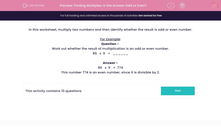Finding Multiples: Is the Answer Odd or Even?

In this worksheet, students multiply two numbers together then state whether the answer is odd or even.This content is premium and exclusive to EdPlace subscribers.Key stage:  KS 2

Curriculum topic:   Maths and Numerical Reasoning

Curriculum subtopic:   Mixed Problems

Difficulty level:Worksheet Overview

In this worksheet, multiply two numbers and then identify whether the result is odd or even number.

For Example:

Question -
Work out whether the result of multiplication is an odd or even number.

86   x  9   =   ______

86   x  9   =  774

This number 774 is an even number, since it is divisible by 2.

What is EdPlace?

We're your National Curriculum aligned online education content provider helping each child succeed in English, maths and science from year 1 to GCSE. With an EdPlace account you’ll be able to track and measure progress, helping each child achieve their best. We build confidence and attainment by personalising each child’s learning at a level that suits them.

Get started